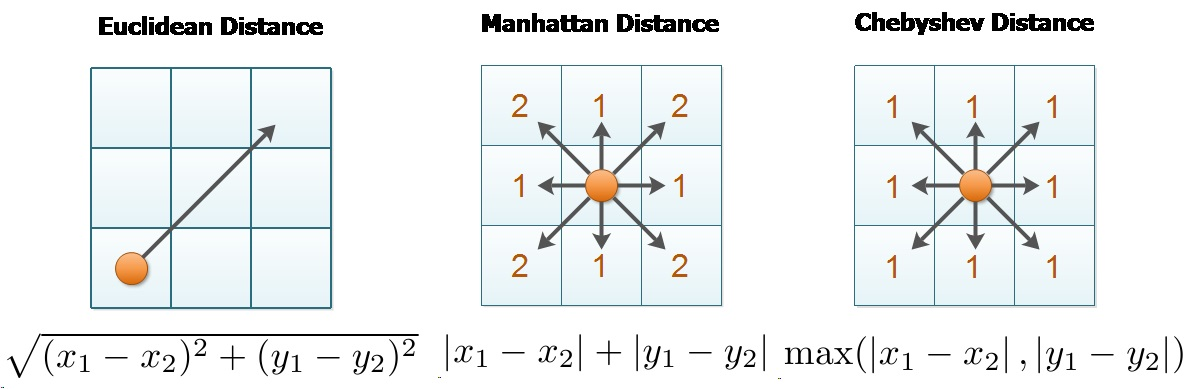×

Search anything:

# Euclidean vs Manhattan vs Chebyshev Distance

#### similarity measurement euclidean distance manhattan distance chebyshev distanceGet this book -> Problems on Array: For Interviews and Competitive Programming

Euclidean distance, Manhattan distance and Chebyshev distance are all distance metrics which compute a number based on two data points. All the three metrics are useful in various use cases and differ in some important aspects which we bring out in this article.

### Visual difference

This image summarizes the difference in the three distance metrics:### Computation

In a N dimensional space, a point is represented as (x1, x2, ..., xN).

Consider two points P1 and P2:


P1: (X1, X2, ..., XN)
P2: (Y1, Y2, ..., YN)


Then, the euclidean distance between P1 and P2 is given as:

$$\sqrt{{(x1-y1)}^2\ +\ {(x2-y2)}^2\ +\ ...\ +\ {(xN-yN)}^2}$$

The manhattan distance between P1 and P2 is given as:

$$|x1-y1|\ +\ |x2-y2|\ +\ ...\ +\ |xN-yN|}$$

The chebyshev distance between the two points P1 and P2 is:


Chebyshev distance = MAXIMUM (|xi - yi|) where i is 1 to N


### Usage in Chess

In chess, all the three distances are used as follows:

• the distance between squares on the chessboard for rooks is measured in Manhattan distance
• kings and queens use Chebyshev distance
• bishops use the Manhattan distance (between squares of the same color) on the chessboard rotated 45 degrees, i.e., with its diagonals as coordinate axes.
• To reach from one square to another, only kings require the number of moves equal to the distance (euclidean distance) rooks, queens and bishops require one or two moves#### OpenGenus Foundation

The official account of OpenGenus IQ backed by GitHub, DigitalOcean and Discourse

Euclidean vs Manhattan vs Chebyshev Distance